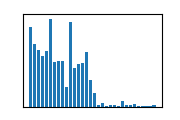# Connected component labeling on surfaces#

This notebook demonstrates how to differentiate objects according to their connectivity.

import napari_process_points_and_surfaces as nppas
import pyclesperanto_prototype as cle
import napari_simpleitk_image_processing as nsitk
from skimage.data import cells3d
import stackview


We use a 3D image of nuclei…

image = cells3d()[:,1]
stackview.insight(image)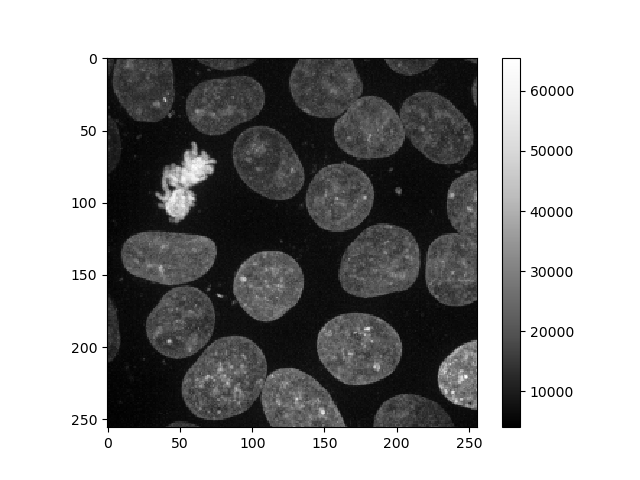shape (60, 256, 256) dtype uint16 size 7.5 MB min 0 max 65535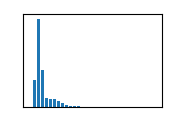… and segment the nuclei resulting in a 3D binary image.

labels = cle.voronoi_otsu_labeling(image, spot_sigma=9)
binary = cle.erode_labels(labels) > 0

stackview.insight(binary)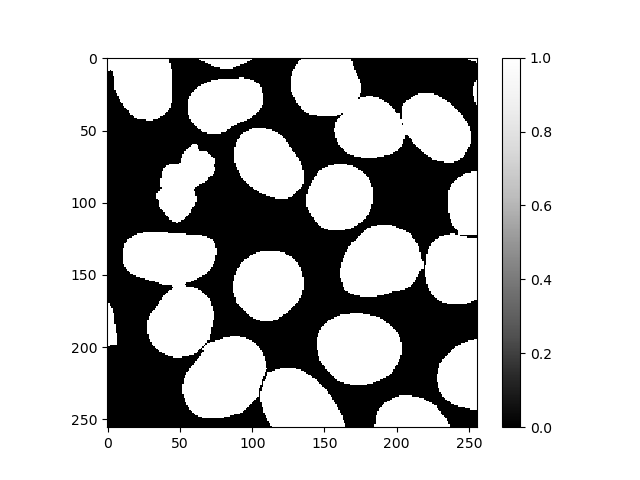shape (60, 256, 256) dtype uint8 size 3.8 MB min 0 max 1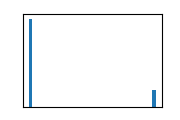We convert this binary image into a surface dataset.

surface = nppas.all_labels_to_surface(binary)
surface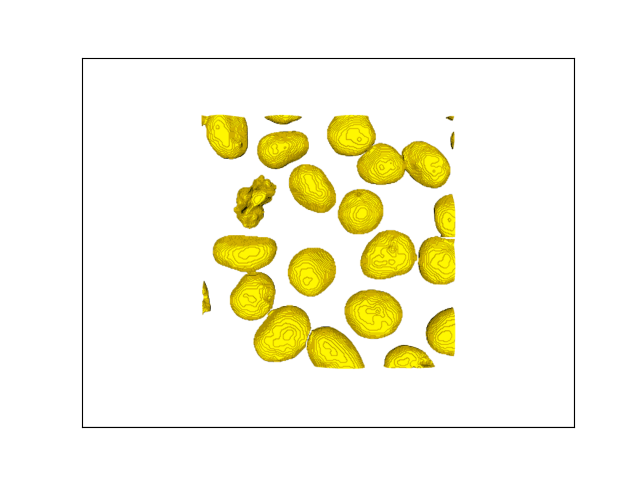nppas.SurfaceTuple
 origin (z/y/x) [0. 0. 0.] center of mass(z/y/x) 34.703,124.973,131.513 scale(z/y/x) 1.000,1.000,1.000 bounds (z/y/x) 16.500...59.0000.000...255.0000.000...255.000 average size 97.003 number of vertices 151354 number of faces 301006

By applying connected component labeling to the surface, we can identify vertices/faces that are connected and differentiate those which are not. The result is also a surface dataset where the vertex values correspond to the nth label these objects belong to. Thus, you can conclude from the maximum number of this surface that thereare 38 nuclei in this image.

surface_connected_components = nppas.connected_component_labeling(surface)
surface_connected_components.cmap = 'hsv'
surface_connected_components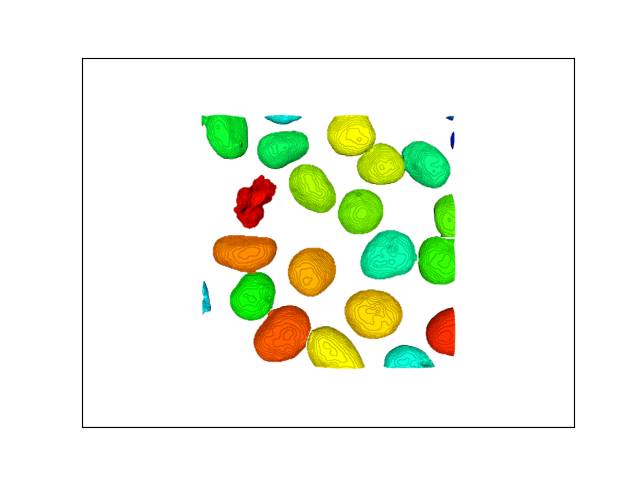nppas.SurfaceTuple
 origin (z/y/x) [0. 0. 0.] center of mass(z/y/x) 34.703,124.973,131.513 scale(z/y/x) 1.000,1.000,1.000 bounds (z/y/x) 16.500...59.0000.000...255.0000.000...255.000 average size 97.003 number of vertices 151354 number of faces 301006 min 0 max 38